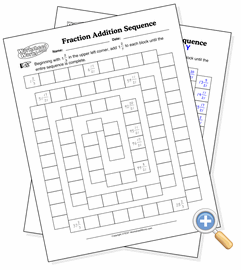# Mixed fraction sequencesAdd mixed fractions in a sequence

This worksheet presents a series of boxes for the student to fill in. You specify the starting mixed fraction and the increment, and the worksheet will prompt for every mixed fraction value in sequence. This is useful for recognizing the addition patterns of fractions and making on-the-spot estimations in more complex formulas.

The starting and incremental fractions have three inputs each: the whole number, the numerator and the denominator. The whole number can be left blank (or zero) to stick with small fractions, but as the values increase in the sequence they will become mixed fractions. The values you enter will determine how difficult this exercise is. Simpler fractions with common denominators (such as one fourth plus one fourth) will of course be easier to add than fractions with higher denominators. Try several combinations to achieve the kind of output your students need.

It is also a good idea to use the “percentage filled” option to randomly have a few boxes filled with the right answer. This helps the student nail down where he or she went wrong in the event that a mistake was made along the way.

Two related math worksheets that use this format are also available: Integer Sequences and Decimal Sequences.